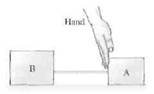# Problem: The hand in the figure is pushing on the back of block A. Blocks A and B, with mB&gt;mA, are connected by a massless string and slide on a frictionless surface. Is the force of the string on B larger than, smaller than, or equal to the force of the hand on A? Explain.

###### FREE Expert Solution

Newton's second law:

$\overline{){\mathbf{\Sigma }}{\mathbf{F}}{\mathbf{=}}{\mathbf{m}}{\mathbf{a}}}$

83% (474 ratings)###### Problem Details

The hand in the figure is pushing on the back of block A. Blocks A and B, with mB>mA, are connected by a massless string and slide on a frictionless surface. Is the force of the string on B larger than, smaller than, or equal to the force of the hand on A? Explain.